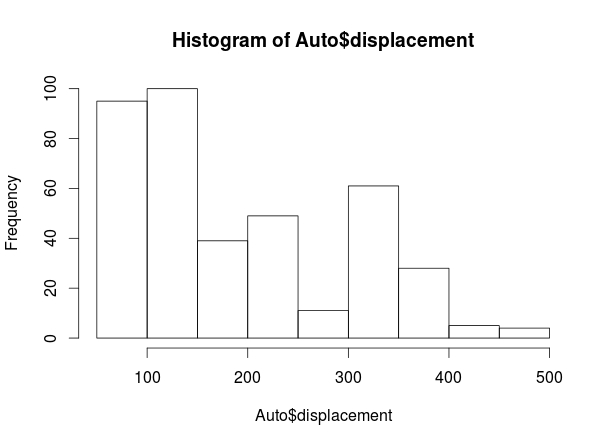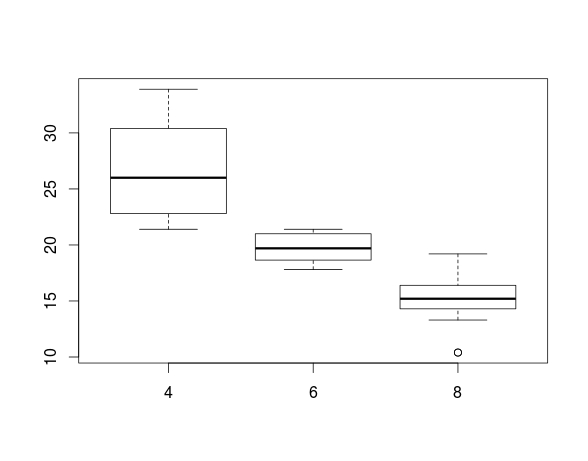# Kruskal-Willis Test in R

Sometimes when the data that needs to be analyzed is not normally distributed. This makes it difficult to make any inferences based on the results because one of the main assumptions of parametric statistical test such as ANOVA, t-test, etc is normal distribution of the data.

Fortunately, for every parametric test there is a non-parametric test. Non-parametric test are test that make no assumptions about the normality of the data. This means that the non-normal data can still be analyzed with a certain measure of confidence in terms of the results.

This post will look at non-parametric test that are used to test the difference in means. For three or more groups we used the Kruskal-Wallis Test. The Kruskal-Wallis Test is the non-parametric version of ANOVA.

Setup

We are going to use the “ISLR” package available on R to demonstrate the use of the Kruskal-Wallis test. After downloading this package you need to load the “Auto” data. Below is the code to do all of this.

```install.packages('ISLR')
library(ISLR)
data=Auto```

We now need to examine the structure of the data set. This is done with the “str” function below is code followed by the results

```str(Auto)
'data.frame':	392 obs. of  9 variables:
\$ mpg         : num  18 15 18 16 17 15 14 14 14 15 ...
\$ cylinders   : num  8 8 8 8 8 8 8 8 8 8 ...
\$ displacement: num  307 350 318 304 302 429 454 440 455 390 ...
\$ horsepower  : num  130 165 150 150 140 198 220 215 225 190 ...
\$ weight      : num  3504 3693 3436 3433 3449 ...
\$ acceleration: num  12 11.5 11 12 10.5 10 9 8.5 10 8.5 ...
\$ year        : num  70 70 70 70 70 70 70 70 70 70 ...
\$ origin      : num  1 1 1 1 1 1 1 1 1 1 ...
\$ name        : Factor w/ 304 levels "amc ambassador brougham",..: 49 36 231 14 161 141 54 223 241 2 ...```

So we have 9 variables. We first need to find if any of the continuous variables are non-normal because this indicates that the Kruskal-Willis test is needed. We will look at the ‘displacement’ variable and look at the histogram to see if it is normally distributed. Below is the code followed by the histogram

```hist(Auto\$displacement)```

This does not look normally distributed. We now need a factor variable with 3 or more groups. We are going to use the ‘origin’ variable. This variable indicates were the care was made 1 = America, 2 = Europe, and 3 = Japan. However, this variable is currently a numeric variable. We need to change it into a factor variable. Below is the code for this

`Auto\$origin<-as.factor(Auto\$origin)`

The Test

We will now use the Kruskal-Wallis test. The question we have is “is there a difference in displacement based on the origin of the vehicle?” The code for the analysis is below followed by the results.

```> kruskal.test(displacement ~ origin, data = Auto)

Kruskal-Wallis rank sum test

data:  displacement by origin
Kruskal-Wallis chi-squared = 201.63, df = 2, p-value < 2.2e-16```

Based on the results, we know there is a difference among the groups. However, just like ANOVA, we do not know were. We have to do a post-hoc test in order to determine where the difference in means is among the three groups.

To do this we need to install a new package and do a new analysis. We will download the “PCMR” package and run the code below

```install.packages('PMCMR')
library(PMCMR)
data(Auto)
attach(Auto)
posthoc.kruskal.nemenyi.test(x=displacement, g=origin, dist='Tukey')```

Here is what we did,

1. Installed the PMCMR package and loaded it
2. Loaded the “Auto” data and used the “attach” function to make it available
3. Ran the function “posthoc.kruskal.nemenyi.test” and place the appropriate variables in their place and then indicated the type of posthoc test ‘Tukey’

Below are the results

```Pairwise comparisons using Tukey and Kramer (Nemenyi) test
with Tukey-Dist approximation for independent samples

data:  displacement and origin

1       2
2 3.4e-14 -
3 < 2e-16 0.51

Warning message:
In posthoc.kruskal.nemenyi.test.default(x = displacement, g = origin,  :
Ties are present, p-values are not corrected.```

The results are listed in a table. When a comparison was made between group 1 and 2 the results were significant (p < 0.0001). The same when group 1 and 3 are compared (p < 0.0001).  However, there was no difference between group 2 and 3 (p = 0.51).

Do not worry about the warning message this can be corrected if necessary

Perhaps you are wondering what the actually means for each group is. Below is the code with the results

```> aggregate(Auto[, 3], list(Auto\$origin), mean)
Group.1        x
1       1 247.5122
2       2 109.6324
3       3 102.7089```

Cares made in America have an average displacement of 247.51 while cars from Europe and Japan have a displacement of 109.63 and 102.70. Below is the code for the boxplot followed by the graph

```boxplot(displacement~origin, data=Auto, ylab= 'Displacement', xlab='Origin')
title('Car Displacement')```Conclusion

This post provided an example of the Kruskal-Willis test. This test is useful when the data is not normally distributed. The main problem with this test is that it is less powerful than an ANOVA test. However, this is a problem with most non-parametric test when compared to parametric test.

# Basics of Histograms and Plots in R

R has many fascinating features for creating histograms and plots. In this post, we will only cover some of the most basic concepts of make histograms and plots in R. The code for the data we are using is available in a previous post.

Making a HistogramWe are going to make a histogram of the ‘mpg’ variable in our ‘cars’ dataset. Below is the code for doing this followed by the actual histogram.

Here is what we did

1. We used the ‘hist’ function to create the histogram
2. Within the hist function we told r to make a histogram of ‘mpg’ variable found in the ‘cars’ dataset.
3. An additional argument that we added was ‘col’. This argument is used to determine the color of the bars in the histogram. For our example, the color was set to gray.

Plotting Multiple Variables

Before we look at plotting multiple variables you need to make an adjustment to the ‘cyl’ variable in our cars variable. THis variable needs t be changed from a numeric to a factor variable as shown below

`cars\$cyl<- as.factor(cars\$cyl)`

Boxplots are an excellent way of comparing groups visually. In this example, we will compare the ‘mpg’ or miles per gallon variable by the ‘cyl’ or number of cylinders in the engine variable in the ‘cars’ dataset. Below is the code and diagram followed by an explanation.

`boxplot(mpg ~ cyl, data = cars)`Here is what happened.

1. We use the ‘boxplot’ function
2. Within this function we tell are to plot mpg and cyl using the tilda  ” ~ ” to tell R to compare ‘mpg’ by the number of cylinders

The box of the boxplot tells you several things

1. The bottom of the box tells you the 25th percentile
2. The line in the middle of the box tells you the median
3. The top of the box tells you the 75th percentile
4. The bottom line tells you the minimum or lowest value excluding outliers
5. The top line tells you the maximum or highest value excluding outliers

In order boxplot above, there are three types of cylinders 4, 6, and 8. For 4 cylinders the 25th percentile is about 23 mpg, the 50th percentile is about 26 mpg, while the 75th percentile is about 31 mpg. The minimum value was about 22 and the maximum value was about 35 mpg. A close look at the different blots indicates that four cylinder cars have the best mpg followed by six and finally eight cylinders.

Conclusions

Histograms and boxplots serve the purpose of describing numerical data in a visual manner. Nothing like a picture helps to explain abstract concepts such mean and median.